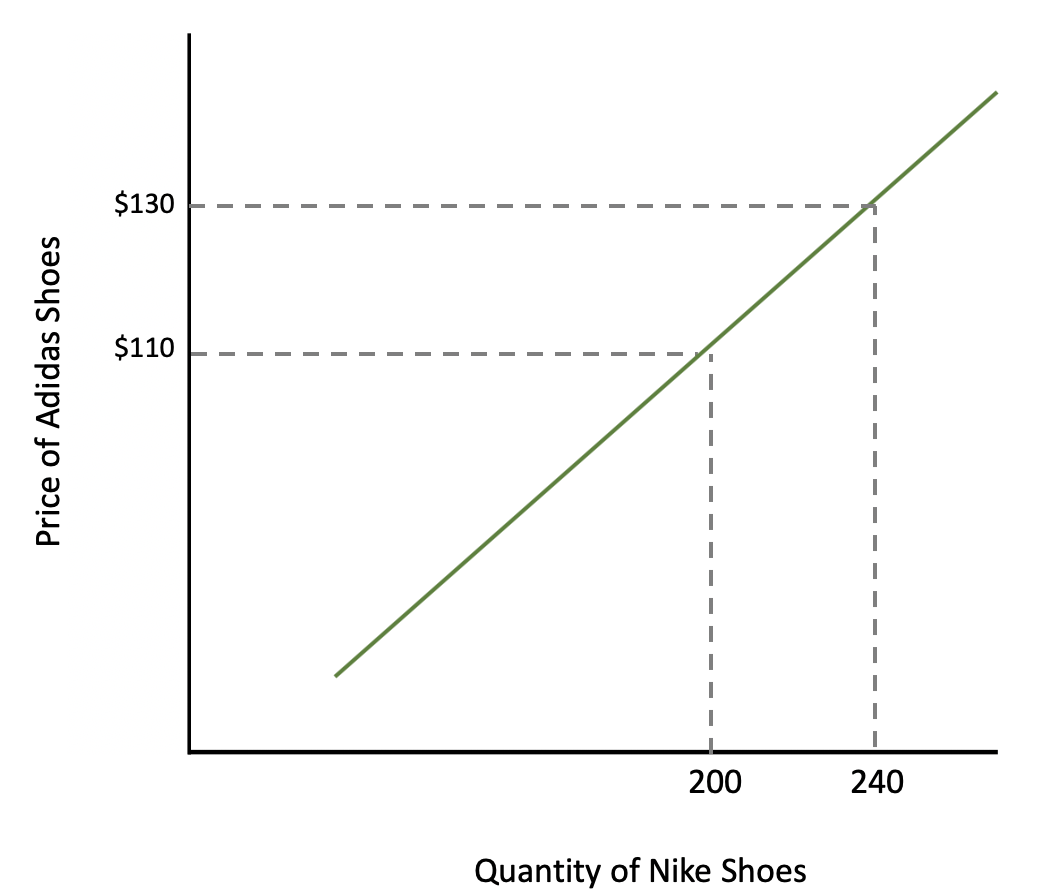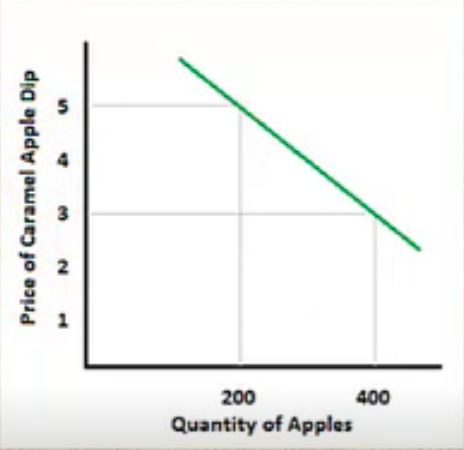+

# Cross-Price Elasticity Formula

Author: Sophia Tutorial
##### Description:

Calculate the cross-price elasticity of two goods.

(more)### Developing Effective Teams

*No strings attached. This college course is 100% free and is worth 1 semester credit.

37 Sophia partners guarantee credit transfer.

299 Institutions have accepted or given pre-approval for credit transfer.

* The American Council on Education's College Credit Recommendation Service (ACE Credit®) has evaluated and recommended college credit for 32 of Sophia’s online courses. Many different colleges and universities consider ACE CREDIT recommendations in determining the applicability to their course and degree programs.

Tutorial
what's covered
This tutorial will cover how to calculate cross-price elasticity when the price of a good changes, as well as discuss the impact on a substitute good and complement good.

Our discussion breaks down as follows:

1. Elasticity of Demand
2. Cross-Price Elasticity
3. Substitute Goods
4. Complement Goods

## 1. Elasticity of Demand

Let's begin with a quick review of the law of demand:

As prices rise, consumers buy less.
As prices fall, consumers buy more.

Elasticity of demand shows how much more or less people respond when the price of a good changes.

To illustrate, let's look at an example using Adidas shoes.

hint
Note, this is own-price elasticity, meaning when the price of something goes up or down, how much more or less do they buy of the good itself?

In today's tutorial, however, we will be discussing how a change in the price of Adidas shoes could impact the sales of something related to it, such as Nike sales.

When Adidas changes price, how could that impact Nike? This refers to cross-price elasticity, so let's begin our discussion of today's topic.

## 2. Cross-Price Elasticity

Cross-price elasticity is defined as the change of demand that occurs due to the change in price of a substitute or complement.

formula
Cross-Price ElasticityEssentially, this formula tells us that elasticity is a proportion. It is the percentage change in quantity, divided by the percentage change in price.

hint
You may recall that when we originally calculated elasticity in a previous tutorial, we calculated the percentage change in quantity and price by taking the respective changes and dividing by the midpoint or average of the two variables. Since this resulted in a "divided by 2" in both the numerator and the denominator, they canceled each other out, leaving us with the simplified formula.

In the case of the cross-price elasticity formula, "Q" refers to the quantity of one good, while "P" refers to the price of another good.

term to know
Cross-Price Elasticity
Change of demand that occurs due to change in price of substitutes or complements

## 3. Substitute Goods

Suppose Adidas decides it is time for them to increase prices on their running shoes. On one model, they raise price from \$110 to \$130.

Certainly, this will impact their sales; their quantity demanded will likely fall as they raise price.

However, what impact will this have on Nike sales?

Suppose Nike sees its sales go from 200 up to 240 this month at a certain store.

Quantity of Nike Shoes Price of Adidas Shoes
QA = 200 PA = \$110
QB = 240 PB = \$130

Here, we are looking at what happens to the quantity of Nike as the price of Adidas shoes changes.Now, this doesn't look like a normal demand curve because we don't have the price of Nike and the quantity of Nike. We have the price of Adidas and its impact on the quantity of Nike.

Let's plug our numbers into the cross-price elasticity equation, using the midpoint formula.

hint
When performing this calculation, it is important to follow the order of operations. The order of QA, QB and PA, PB does matter.So, we end up with a cross-price elasticity coefficient of 1.125. Since the order did matter, it is important to note that this is a positive number.

However, what does this number actually tell us?

Well, it tells us whether the two products are substitute goods or complement goods.

Generally speaking, quantity and price are going to move in opposite directions.

EXAMPLE

As the price of Nike goes up, the quantity that people purchase goes down.

However, with cross-price elasticity:

If the coefficient is positive, then the two goods must be substitutes.
If the coefficient is negative, then the two goods must be complements.

In this case, with an elasticity of 1.125, Nike and Adidas must be substitutes, since the coefficient was positive.

When Adidas raises prices, people will buy more of a substitute like Nike.

Now, they're not perfect substitutes. Some people strongly prefer one to the other, but this reflects the pattern that we determined.

Substitute goods, then, refer to a situation where as the price of one good increases, like Adidas, the demand for an alternative good meeting the same consumer needs, like Nike, increases.

term to know
Substitute Goods
As the price of one good increases, the demand for an alternative good meeting the same consumer needs increases

## 4. Complement Goods

Now let's explore a different situation, looking at the demand for apples.

In this example, we're not going to change the price of apples. Rather, we're going to change the price of caramel apple dip.

Suppose caramel apple dip goes on sale from \$5 to \$3. What will happen to the demand for apples?

Well, let's assume that a grocery stores sees apple sales increase from a quantity of 200 to 400.

Here is our graph. Remember, we are concerned with the effect on the quantity of apples purchased, but the price on the y-axis is not of apples--it's of caramel apple dip. Therefore, we are looking at the effect on the quantity of apples demanded as the price of caramel apple dip changes.Again, remember that the order of operations does matter.So, with an elasticity of negative 1.32, apples and caramel dip must be complement goods, since the coefficient is negative.

When caramel apple dip goes on sale, people will buy more apples to go with it.

This is exactly what complements are--they are goods for which the demand increases as the price of an associated good decreases.

As caramel apple dip went on sale, people bought the complement more, and the demand for apples increased.

term to know
Complement Goods
A good for which the demand increases as the price of an associated good decreases

summary
We began today's tutorial with a brief review of elasticity of demand. We learned that cross-price elasticity measures how consumers respond to a price change by buying a related good, as opposed to the actual good itself, which is own-price elasticity. We learned that the elasticity coefficient will tell us how much consumers respond and whether the goods involved are substitute goods or complement goods. Remember if you get a positive coefficient, it means the two goods are substitutes. If you get a negative coefficient, it indicates that the two goods are complements.

Source: Adapted from Sophia instructor Kate Eskra.

Terms to Know
Complement Goods

A good for which the demand increases as the price of an associated good decreases.

Cross-Price Elasticity

Change of demand that occurs due to change in price of substitutes or complements.

Substitute Goods

As the price of one good increases, the demand for an alternative good meeting the same consumer needs increases.

Formulas to Know
Cross-Price ElasticityRating